In Online Education NCERT Solutions for Class 8 Maths Chapter 8 Comparing Quantities Ex 8.3 are part of NCERT Solutions for Class 8 Maths. Here we have given NCERT Solutions for Class 8 Maths Chapter 8 Comparing Quantities Ex 8.3.

 Board CBSE Textbook NCERT Class Class 8 Subject Maths Chapter Chapter 8 Chapter Name Comparing Quantities Exercise Ex 8.3 Number of Questions Solved 12 Category NCERT Solutions

## Online Education NCERT Solutions for Class 8 Maths Chapter 8 Comparing Quantities Ex 8.3

Question 1.
Calculate the amount and compound interest on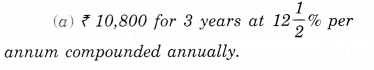Solution.
(a) By using year by year calculation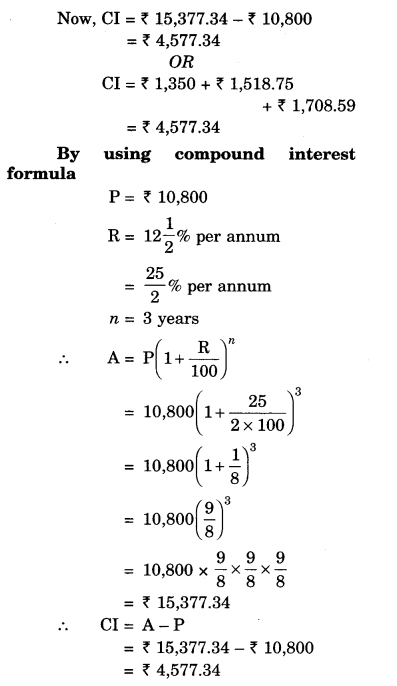(b) By using year by year calculation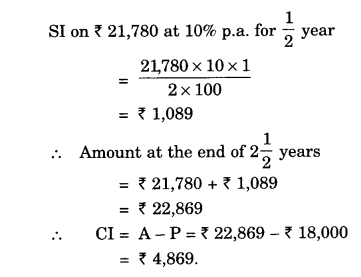(c) By using half year by half year calculation(d) By using half-year by half-year calculation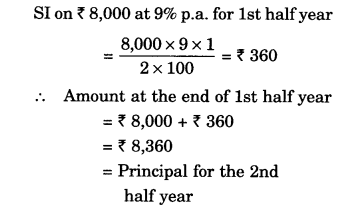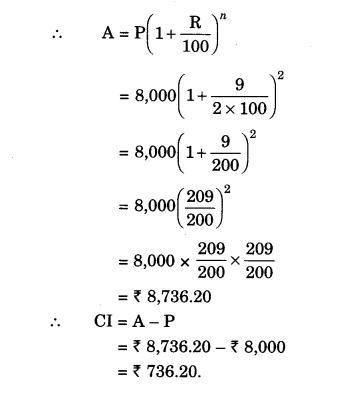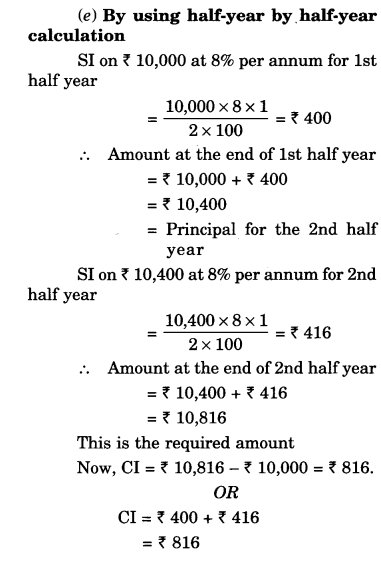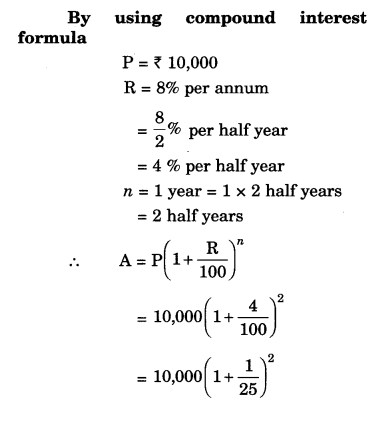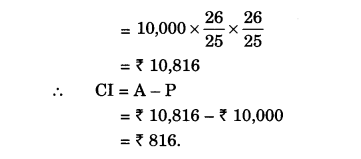Question 2.
Kamala borrowed ₹ 26,400 from a Bank to buy a scooter at a rate of 15% p.a. compounded yearly. What amount will she pay at the end of 2 years and 4 months to clear the loan?
(Hint: Find A for 2 years if interest is compounded yearly and then find SI on the 2nd year amount for $$\frac { 4 }{ 12 }$$ year)
Solution.Question 3.
Fabina borrows ₹ 12,500 at 12% per annum for 3 years at simple interest and Radha borrows the same amount for the same time period at 10% per annum, compounded annually. Who pays more interest and by how much ?
Solution.
For Fabina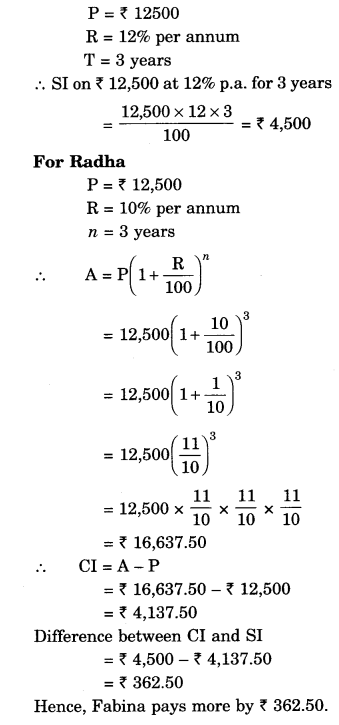Question 4.
I borrowed ₹ 12,000from Jamshed at 6% per annum simple interest for 2 years. Had I borrowed this sum at 6% per annum compound interest, what excess amount would I have to pay?
Solution.
At simple interest
P = ₹ 12000
R = 6% per annumQuestion 5.
Vasudevan invested ₹ 60,000 on interest at the rate of 12% per annum compounded half yearly. What amount would he get
(i) after 6 months?
(ii) after 1 year?
Solution.
(i) after 6 months
P = ₹ 60,000
R = 12% per annum(ii) after 1 yearQuestion 6.
Arif took a loan of ₹ 80,000 from a bank. If the rate of interest is 10% per annum, find the difference in amounts he would be paying after $$1\frac { 1 }{ 2 }$$ years if the interest is
(i) compounded annually
(ii) compounded half yearly
Solution.
(i) compounded annually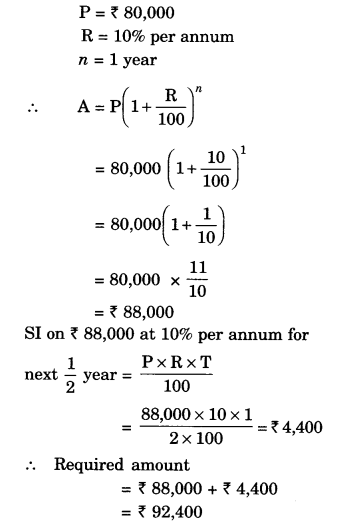(ii) compounded half yearly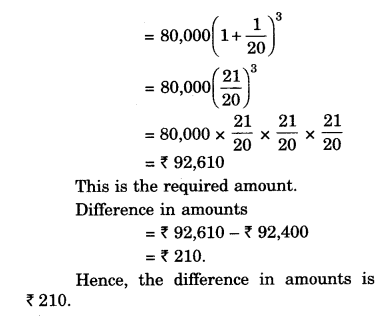Question 7.
Maria invested ₹ 8,000 in business. She would be paid interest at the rate of 5% per annum compounded annually. Find
(i) the amount credited against her name at the end of the second year.
(ii) the interest for the 3rd year.
Solution.
(i)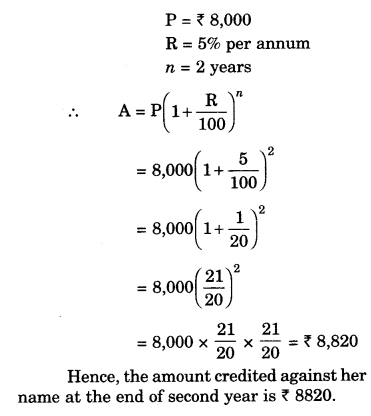(ii)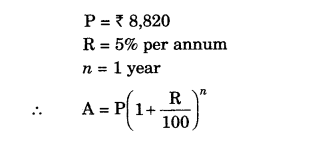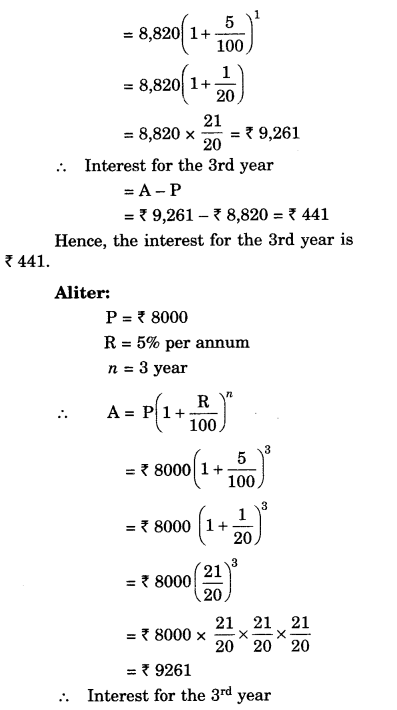Question 8.
Find the amount and the compound interest on ₹ 10,000 for $$1\frac { 1 }{ 2 }$$ years at 10% per annum, compounded half yearly. Would this interest be more than the interest he would get if it was compounded annually?
Solution.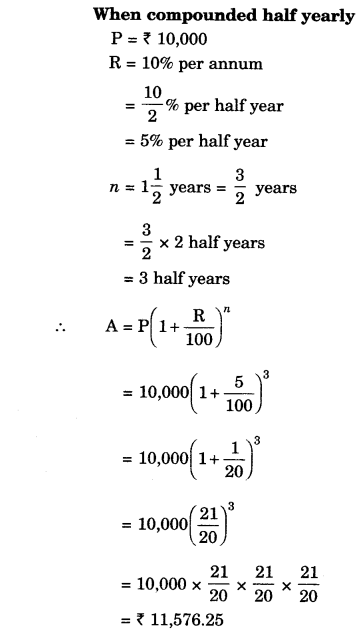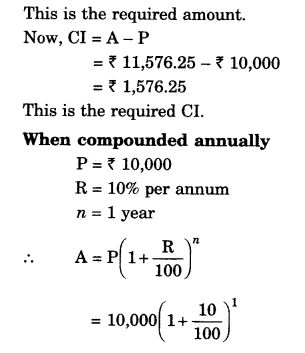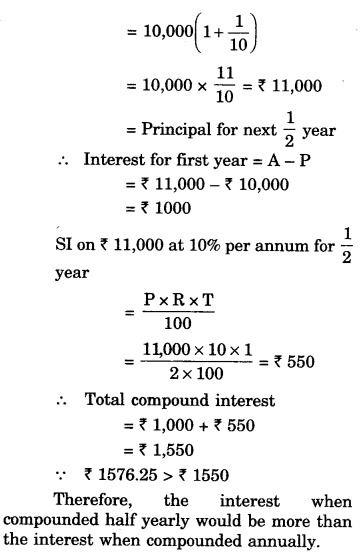Question 9.
Find the amount which Ram will get on ₹ 4,096 if he gave it for 18 months at $$12\frac { 1 }{ 2 } %$$ per annum, interest being compounded half yearly.
Solution.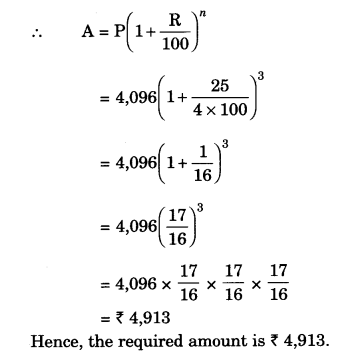Question 10.
The population of a place increased to 54,000 in 2003 at a rate of 5% per annum.
(i) find the population in 2001.
(ii) what would he its population in 2005?
Solution.
(i)
Let the population in 2001 be P.
R = 5% p.a.
n = 2 years(ii)
initial population in 2003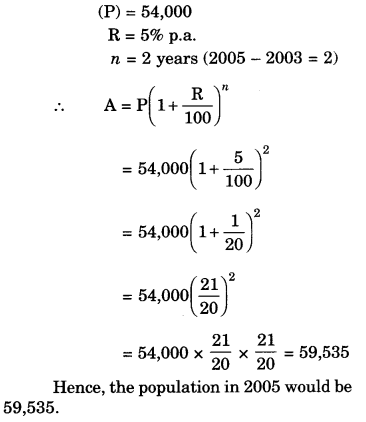Question 11.
In a Laboratory, the count of bacteria in a certain experiment was increasing at the rate of 2.5% per hour. Find the bacteria at the end of 2 hours, if the count was initially 5,06,000.
Solution.
Initial count of bacteria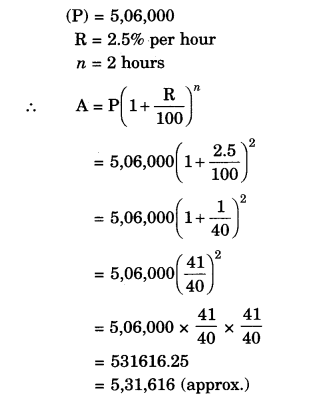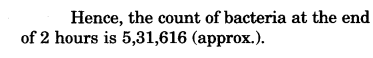Question 12.
A scooter was bought at ₹ 42,000. It’s value depreciated at the rate of 8% per annum. Find its value after one year.
Solution.
Initial value of the scooter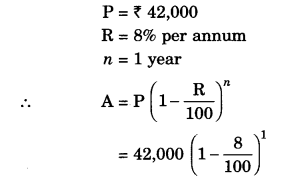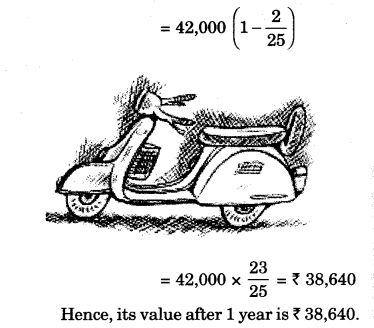We hope the NCERT Solutions for Class 8 Maths Chapter 8 Comparing Quantities Ex 8.3 help you. If you have any query regarding NCERT Solutions for Class 8 Maths Chapter 8 Comparing Quantities Ex 8.3, drop a comment below and we will get back to you at the earliest.